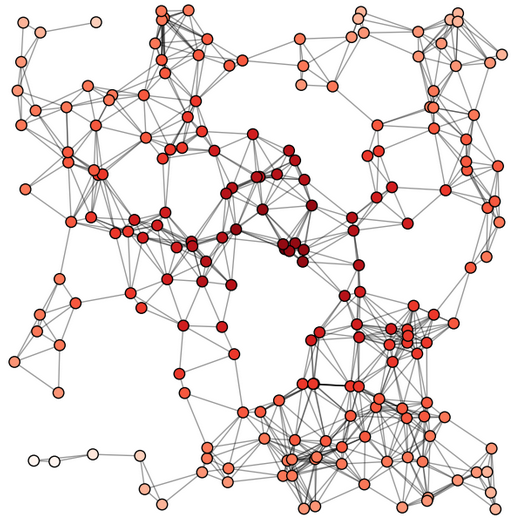# python—networkx：画随机几何图，找出中心节点并按路径长度染色

• 随机生成一个集合图，自带路径属性，图大小为1*1，找出距离中心[0.5, 0.5]最近的节点，并按照路径染色。
• NetworkX Examples » Drawing » Random Geometric Graph

<pre name="code" class="python">#coding:utf-8
import networkx as nx
import matplotlib.pyplot as plt

G=nx.random_geometric_graph(200,0.125)
# position is stored as node attribute data for random_geometric_graph
pos=nx.get_node_attributes(G,'pos')
# find node near center (0.5,0.5)找到中心节点并求最近的节点，设为ncenter
dmin=1
ncenter=0
for n in pos:
x,y=pos[n]
d=(x-0.5)**2+(y-0.5)**2
if d<dmin:
ncenter=n
dmin=d
# color by path length from node near center颜色定为红色，程度<span style="font-family: Arial, Helvetica, sans-serif;">按距离中心点的路径长度染色</span>
p=nx.single_source_shortest_path_length(G,ncenter)
plt.figure(figsize=(8,8))
nx.draw_networkx_edges(G,pos,nodelist=[ncenter],alpha=0.4)
nx.draw_networkx_nodes(G,pos,nodelist=p.keys(),
node_size=80,
node_color=p.values(),
cmap=plt.cm.Reds_r)
plt.xlim(-0.05,1.05)
plt.ylim(-0.05,1.05)
plt.axis('off')
plt.savefig('random_geometric_graph.png')
plt.show()©️2019 CSDN 皮肤主题: 编程工作室 设计师: CSDN官方博客